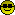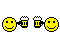###Author Topic: voxel waves  (Read 1165 times)

0 Members and 1 Guest are viewing this topic.

####boogop

• C= 64
•• Posts: 66
• Karma: 42##### voxel waves
« on: April 04, 2018 »
Does anyone know of or have an example of this effect, the one that future crew had in second reality? The only example I've been able to find is by Insolit Dust who used to post here, but it's difficult to read and has enough hardcoded values that I can't really tell what it's trying to do. Any language should be okay, but it's more difficult for me to deconstruct an xy location if the video is addressed like unsigned char *b = DBuf + (a <<+ (a << 6) + i;

snippet from insolit dust, he posted a link to his site here so I hope this is okay!

Code: [Select]
`void Line(int x0, int y0, int x1, int y1, int hy, int s){ register int i;     int sx, sy;     int c,y,h,u0,v0,u1,v1,a,b,h0,h1,h2,h3;          sx = (x1 - x0) / 320;     sy = (y1 - y0) / 320;          for (i = 0; i < 320; i++)     {         u0 = (x0 >> 16) & 0xFF;         a = (x0 >> 8) & 255;         v0 = ((y0 >> 8) & 0xFF00);         b = (y0 >> 8) & 255;         u1 = (u0 + 1) & 0xFF;         v1 = (v0 + 256) & 0xFF00;                  h0 = HMap[u0 + v0];         h2 = HMap[u0 + v1];         h1 = HMap[u1 + v0];         h3 = HMap[u1 + v1];                  h0 = (h0 << 8) + a * (h1 - h0);         h2 = (h2 << 8) + a * (h3 - h2);         h = ((h0 << 8) + b * (h2 - h0)) >> 16;                  h0 = CMap[u0 + v0];         h2 = CMap[u0 + v1];         h1 = CMap[u1 + v0];         h3 = CMap[u1 + v1];                  h0 = (h0 << 8) + a * (h1 - h0);         h2 = (h2 << 8) + a * (h3 - h2);         c = ((h0 << 8) + b * (h2 - h0));                  y = (((h - hy) * s) >> 11) + (240 / 2);                  if (y < (a = LastY[i]))         {                unsigned char *b = DBuf + (a << 8) + (a << 6) + i;                int sc,cc;                                if (LastC[i] == -1)                   LastC[i] = c;                                   sc = (c - LastC[i]) / (a - y);                cc = LastC[i];                                if (a > 240 - 1)                {                      b -= (a - (240 - 1)) * 320;                      cc += (a - (240 - 1)) * sc;                      a = 240 - 1;                }                                if (y < 0)                   y = 0;                                   while (y < a)                {                      *b = cc >> 18;                      cc += sc;                      b -= 320;                      a--;                }                                LastY[i] = y;         }                  LastC[i] = c;                  x0 += sx;         y0 += sy;     }}`

####inc.##### Re: voxel waves
« Reply #1 on: April 04, 2018 »
Can you upload a picture by this effect?  I think i did this back in the days on PlayStation 1 in some Cracktros.
If it is the same,  i can provide code

Cheers
currently coding in PureBasic: GLSL Shader Maker & Editor Tool for further Demo coding usage

####boogop

• C= 64
•• Posts: 66
• Karma: 42##### Re: voxel waves
« Reply #2 on: April 05, 2018 »
Attached.  I haven't seen it done a lot, and in the case of insolit  dust's code it would probably make more sense if you'd done a lot of stuff similarly complex. Future crew's source is illegible lol

####hellfire

• Pentium
••• Posts: 1289
• Karma: 466##### Re: voxel waves
« Reply #3 on: April 06, 2018 »
The given source code seems to draw a perspective floor (which is basically a rotozoomer with different scaling in every scanline).
But when you draw a pixel, you draw a vertical line instead.
The height of that line is fetched from the texture "HMap" (a greyscale heightmap, white=255=high, black=0=flat).
Because that would create a lot of overdraw, you track the current height of each screen column in "LastY[]" (this means you have to fill the screen bottom to top = front to back).
So if you have already filled a column up to line 100 and your next line goes up to 90, you only have to fill the remaining 10 pixels at the top because the lower part is occluded.

The screen resolution (320x240) and the texture size (256x256) are hardcoded (that's the bitmasking with FF).
u0/u1, v0/v1 are used to fetch a 2x2 set of texels for bilinear filtering ("a" and "b" are the 8bit-subpixel components of x0, y0).

The color of each pixel is the interpolated height "cc" so all lines get a gradient from dark (at the bottom) to bright (at the top).

This:
Code: [Select]
`(a << 8) + (a << 6)`is used to avoid a multiply by 320 which doesn't make so much sense since 20 years.
« Last Edit: April 06, 2018 by hellfire »

####Rbz

• Founder Member
••• Posts: 2721
• Karma: 485##### Re: voxel waves
« Reply #4 on: April 06, 2018 »
Excellent explanation Hellfire!
Thanks####boogop

• C= 64
•• Posts: 66
• Karma: 42##### Re: voxel waves
« Reply #5 on: April 07, 2018 »
wow, I actually understood that! I don't know that I'm smart enough to reproduce it, but I have a better sense of what the code's doing. Karma coming your way! =:D